# ISEE Middle Level Quantitative : How to divide fractions

## Example Questions

2 Next →

### Example Question #11 : Fractionsis a positive number, and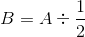. Which is the greater quantity?

(a) The reciprocal of(b) The reciprocal of(a) is the greater quantity

(a) and (b) are equal

(b) is the greater quantity

It is impossible to determine which quantity is the greater from the information given

(a) is the greater quantity

Explanation: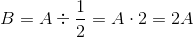.

The reciprocal ofis therefore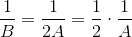- that is,times the reciprocal of. The reciprocal of a positive number must be positive, and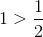, so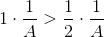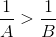The reciprocal ofis the greater number.

### Example Question #12 : Fractions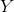is a positive number. Which is the greater quantity?

(a) The reciprocal of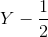(b) The reciprocal of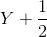(b) is the greater quantity

(a) and (b) are equal

(a) is the greater quantity

It is impossible to determine which is greater from the information given

It is impossible to determine which is greater from the information given

Explanation:

We examine two scenarios to demonstrate that the given information is insufficient.

Case 1: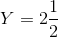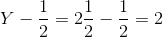The reciprocal of this is.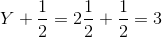The reciprocal of this is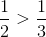, sohas the greater reciprocal.

Case 2: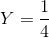.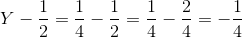The reciprocal of this is.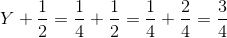The reciprocal of this is.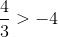, sohas the greater reciprocal.

### Example Question #13 : Fractionsis a negative number. Which is the greater quantity?

(a) The reciprocal of(b) The reciprocal of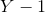It is impossible to determine which is greater from the information given

(a) is the greater quantity

(a) and (b) are equal

(b) is the greater quantity

(b) is the greater quantity

Explanation:is negative, so we will let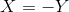, makingpositive. It follows that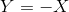.

The reciprocal ofis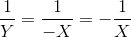;

the reciprocal ofis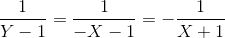.and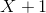are both positive;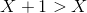, so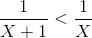, and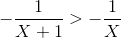. Therefore, the reciprocal ofis the greater quantity.

2 Next →